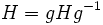# Weakly closed implies conjugation-invariantly relatively normal in finite group

## Statement

Suppose$H \le K \le G$ are groups such that$H$ is a Weakly closed subgroup (?) of$K$ relative to$G$. Then,$H$ is a Conjugation-invariantly relatively normal subgroup (?) of$K$ relative to$G$, viz.,$H$ is normal in every conjugate of$K$ in$G$ containing it.

## Facts used

1. Weakly closed implies normal in middle subgroup

## Proof

Given: Groups$H \le K \le G$ such that$H$ is weakly closed in$K$ with respect to$G$.

To prove: If$g \in G$ is such that$H \le gKg^{-1}$, then$H$ is normal in$K$.

Proof:

1.$g^{-1}Hg \le H$: Since$H \le gKg^{-1}$, we have$g^{-1}Hg \le K$. Now, since$H$ is weakly closed in$K$, we get that$g^{-1}Hg \le H$.
2.$g^{-1}Hg = H$: Since$G$ is finite, and conjugation by$g$ is an automorphism, the sizes of$g^{-1}Hg$ and$H$ are the same. This, along with the previous step, yields$g^{-1}Hg = H$.
3.$H = gHg^{-1}$: This follows from the previous step by conjugating both sides by$g$.
4.$H$ is weakly closed in$gKg^{-1}$: Since$H$ is weakly closed in$K$, and conjugation by$g$ is an automorphism,$gHg^{-1}$ is weakly closed in$gKg^{-1}$.$H = gHg^{-1}$ by the previos step, so$H$ is weakly closed in$gKg^{-1}$.
5.$H$ is normal in$gKg^{-1}$: This follows from the previous step, and fact (1).